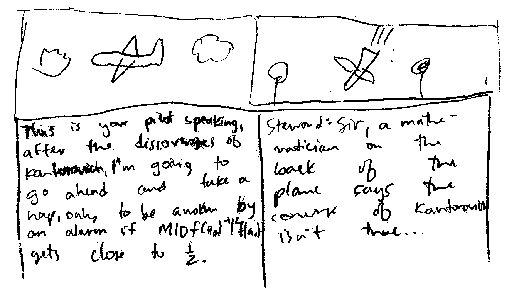MainPresentationsPicturesNotesTeaching

## Miscellaneous notes

These are notes I hope may be useful. Please let me know if you find any errors.

Slides from conference lecture in Roscoff, France.

Complex structures on a vector space
Siegel half-plane
Conformal metrics and hyperbolic geometry (lecture notes for one class of complex analysis, Math 612) Thanks to Tim Goldberg for helpful discussions about curvature, both Gaussian and geodesic.
Čech cohomology and de Rham's theorem (lecture notes for two classes of symplectic geometry, Math 758)

I have written some introductory notes on LaTeX.

## Notes for Math 223, Fall 2005

This is an advanced course for beginning math majors; the topics are introductory linear algebra, multivariable calculus, and manifold theory. The textbook is by John Hubbard, and the instructor for the course at the time I made these notes was Todd Kemp. (I was the teaching assistant.)

Complex numbers pdf dvi
Open sets, closed sets, and continuity pdf dvi
Euler's identity and derivatives pdf dvi
Limits of rational functions pdf dvi
Geometry of systems of linear equations pdf dvi
Fundamental Theorem of Linear Algebra, and graphing pdf dvi
Abstract vector spaces (class lecture) pdf dvi
Newton's method pdf dvi
Inverse Function Theorem pdf dvi
Manifolds pdf dvi
Proof of Taylor's Theorem for real-valued Ck-functions of several variables pdf dvi
Taylor's theorem with tensors pdf dvi
Gaussian curvature of surfaces pdf dvi

### My “eigenfaces”

Created by math 223 student Alex Gates for his paper on the spectral theorem, using my directory photo.### Kantorovich cartoon

Written by math 223 student Kevin Poenisch, on the occasion of the final.Captions:
“This is your pilot speaking. After the discoveries of Kantorovich, I’m going to go ahead and take a nap, only to be awoken by an alarm if M | Df (a0)-1 |2 f (a0) gets close to 1/2.”
Steward: “Sir, a mathematician on the back of the plane says the converse of Kantorovich isn’t true…”

Kantorovich’s Theorem gives conditions that guarantee convergence of Newton’s method to a root of a given C1 function f. (The plane analogy is that the plane’s height is the function value; for the plane, “converging to a root” means crashing to the ground.) It states that, if the above expression has a value less than or equal to 1/2, then the function has a unique root in a certain ball (determined by the first step of Newton’s method), and that Newton’s method will converge to it. (M is a Lipschitz constant for the derivative, ensuring that it doesn’t change too quickly.)